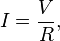## 2013/10/30

### Decoding Les Physz

This page is dedicated to those who does not get the following comic strip:
-- Source Credit

It is basically Les Misérables à la physics.

Schrödinger Equation:

In quantum mechanics, the Schrödinger equation is a partial differential equation that describes how the quantum state of some physical system changes with time. It was formulated in late 1925, and published in 1926, by the Austrian physicist Erwin Schrödinger.
In classical mechanics, the equation of motion is Newton's second law, and equivalent formulations are the Euler–Lagrange equations and Hamilton's equations. All of these formulations are used to solve for the motion of a mechanical system and mathematically predict what the system will do at any time beyond the initial settings and configuration of the system.

Les Miserables (Original London Cast 1985) - I Dreamed a Dream:

Dipole Magnet:

dipole magnet is an electromagnet used in particle accelerators to create a homogeneous magnetic field over some distance. Particle motion in that field will be circular in a plane perpendicular to the field and collinear to the direction of particle motion and free in the direction orthogonal to it. Thus, a particle injected into a dipole magnet will travel on a circular or helical trajectory. By adding several dipole sections on the same plane, the bending radial effect of the beam increases.

Les Misérables-Do You Hear The People Sing:

Empty Charts of Empty Data vs Les Miserables - Empty Chairs at Empty Tables:

Ohm's Law:

Ohm's law states that the current through a conductor between two points is directly proportional to the potential difference across the two points. Introducing the constant of proportionality, the resistance, one arrives at the usual mathematical equation that describes this relationship:$I = \frac{V}{R},$
where I is the current through the conductor in units of amperesV is the potential difference measured across the conductor in units of volts, and R is the resistance of the conductor in units of ohms. More specifically, Ohm's law states that the R in this relation is constant, independent of the current.

Les Misérables - On My Own (2012):

Californium-251:

Californium is a radioactive metallic chemical element with the symbol Cf and atomic number 98. The element was first made at theUniversity of California, Berkeley in 1950 by bombarding curium with alpha particles (helium-4 ions). It is an actinide element, the sixthtransuranium element to be synthesized, and has the second-highest atomic mass of all the elements that have been produced in amounts large enough to see with the unaided eye (after einsteinium). The element was named after California and the University of California. It is the heaviest element to occur naturally on Earth; heavier elements can only be produced by synthesis.
Two crystalline forms exist for californium under normal pressure: one above 900 °C and one below 900 °C. A third form exists at high pressure. Californium slowly tarnishes in air at room temperature. Compounds of californium are dominated by a chemical form of the element, designated californium(III), that can participate in three chemical bonds. The most stable of californium's twenty known isotopes is californium-251, which has a half-life of 898 years. This short half-life means the element is not found in significant quantities in the Earth's crust.[a] Californium-252, with a half-life of about 2.64 years, is the most common isotope used and is produced at the Oak Ridge National Laboratory in the United States and the Research Institute of Atomic Reactors in Russia.

Les Misérables - Who Am I? 24601!

Encore: Do You Hear the People Sing à la Fromosan

Related Article: